# Praxis Mathematics: Trigonometry Chapter Exam

Exam Instructions:

Choose your answers to the questions and click 'Next' to see the next set of questions. You can skip questions if you would like and come back to them later with the yellow "Go To First Skipped Question" button. When you have completed the practice exam, a green submit button will appear. Click it to see your results. Good luck!

### Page 1

#### Question 1 1. Solve the following trigonometric equation for x.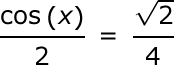#### Question 2 2. What's the value of 2sin(θ)cos(θ) given that the equation below is true?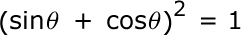#### Question 3 3. Solve for the value of x in the trigonometric equation below. (Use the domain -π/2 to π/2)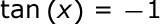#### Question 4 4. In the pictured triangle, ∠A is 137 degrees and ∠B is 28 degrees. If side b is 71 units long, approximately how long is side a?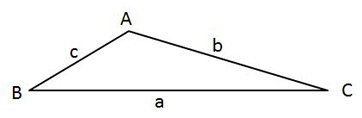### Page 2

#### Question 6 6. Simplify the following expression. Report the answer in degrees.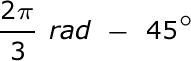#### Question 7 7. Solve the following trigonometric equation for θ.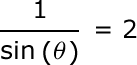#### Question 8 8. In the pictured triangle, side a is 23, side b is 18, and side c is 16. What is the approximate measure of ∠C? When calculating the inverse cosine, round the initial value to the nearest whole number.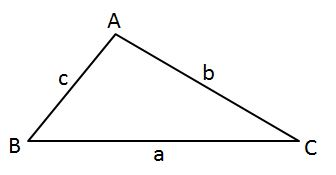#### Question 9 9. Find the value of x, rounded to the nearest tenth.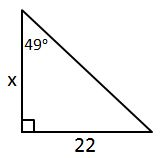#### Question 10 10. What is the value of cos(θ) given that the equation below is true?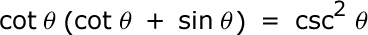### Page 3

#### Question 11 11. Find the value of x, rounded to the nearest degree.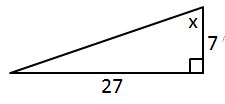#### Question 12 12. In the pictured triangle, ∠A is 98 degrees and ∠B is 12 degrees. If side a is 84 units long, approximately how long is side b?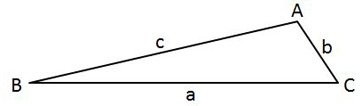#### Question 13 13. In the pictured triangle, what ratio represents the cosine of theta?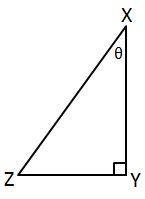#### Question 15 15. In the pictured triangle, ∠A is 62 degrees and ∠C is 86 degrees. If side a is 112 units long, approximately how long is side c?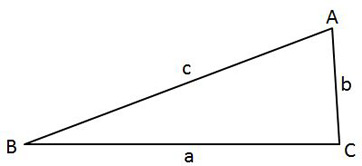### Page 4

#### Question 16 16. Find the value of x, rounded to the nearest degree.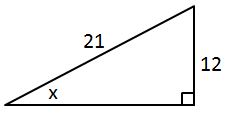#### Question 17 17. What is x?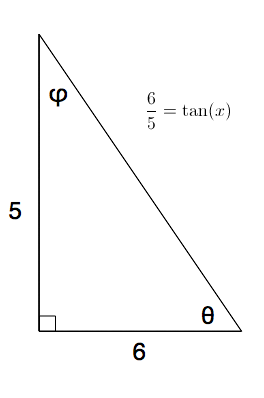#### Question 18 18. What's the value of sec2 (θ) given that the equation below is true?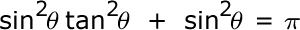#### Question 20 20. Simplify the following expression.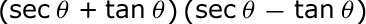### Page 5

#### Question 21 21. The lines a and b are parallel lines of length 5 and 2. The lines d and e are parallel to one another, and both are perpendicular to a and b. What is the length of c?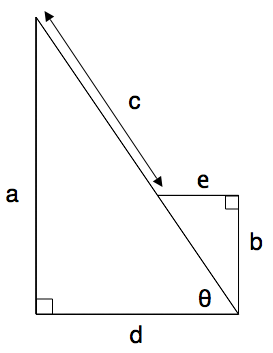#### Question 22 22. In the pictured triangle, side a is 9 and side b is 6. If ∠C is 26 degrees, then approximately how long is side c? Round the value of the cosine to the nearest whole number.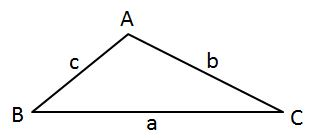#### Question 23 23. Simplify the following expression.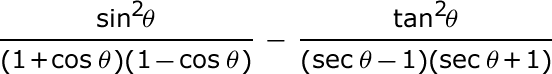#### Question 24 24. In the pictured triangle, side a is 17 and side c is 20. If ∠B is 19 degrees, then approximately how long is side b? Round the value of the cosine to the nearest whole number.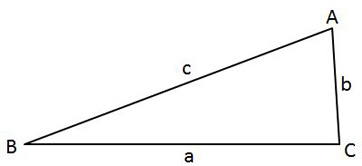#### Question 25 25. Solve the following equation for θ.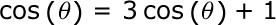### Page 6

#### Question 26 26. In the pictured triangle, ∠A is 58 degrees and ∠C is 63 degrees. If side a is 84 units long, approximately how long is side c?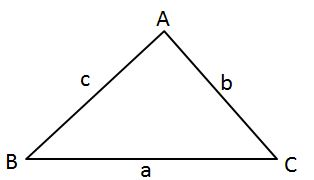#### Question 28 28. Find the value of x, rounded to the nearest tenth.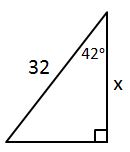#### Question 29 29. Perform the following calculation. Report the answer in radians.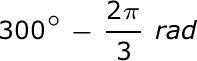#### Praxis Mathematics: Trigonometry Chapter Exam Instructions

Choose your answers to the questions and click 'Next' to see the next set of questions. You can skip questions if you would like and come back to them later with the yellow "Go To First Skipped Question" button. When you have completed the practice exam, a green submit button will appear. Click it to see your results. Good luck!

Support